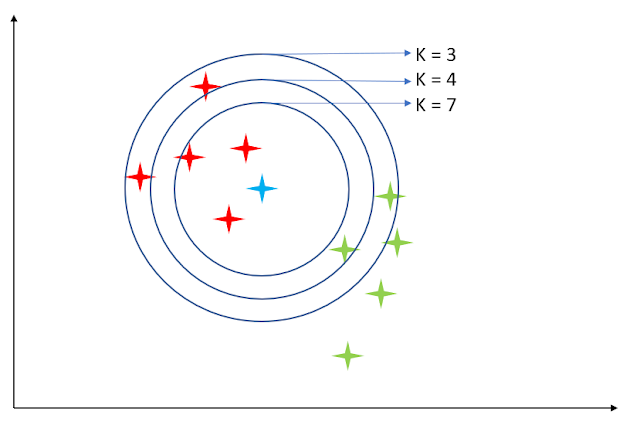## Posts

Showing posts from November, 2019

### kNN Algorithm Python ImplementationkNN is for k Nearest Neighbour.
kNN can be used as a classification algorithm as well as the regression algorithm. I am applying kNN for this project as a classification algorithm. kNN algorithm chooses k nearest neighbors of the test data and then classifies the test data to that class which has the highest frequency among the k nearest neighbors.
In simple words, let us take an example of the below image

We have 5 red plots and 5 green plot in the coordinate system. Now, we have test data which has been shown as the blue plot. Our task is to predict the class of the blue plot. We will now calculate the euclidean distance of the blue plot from other plots. The value of K suggests the number of nearest points that we have to consider. For K = 3, we select the 3 nearest (lowest to highest Euclidean Distance) neighbors. We have 3 plots. Most of the plots belong to class "Red", thus the test data belongs to class "Red".
Now, choosing K = 4,  we select the 4 nearest (…